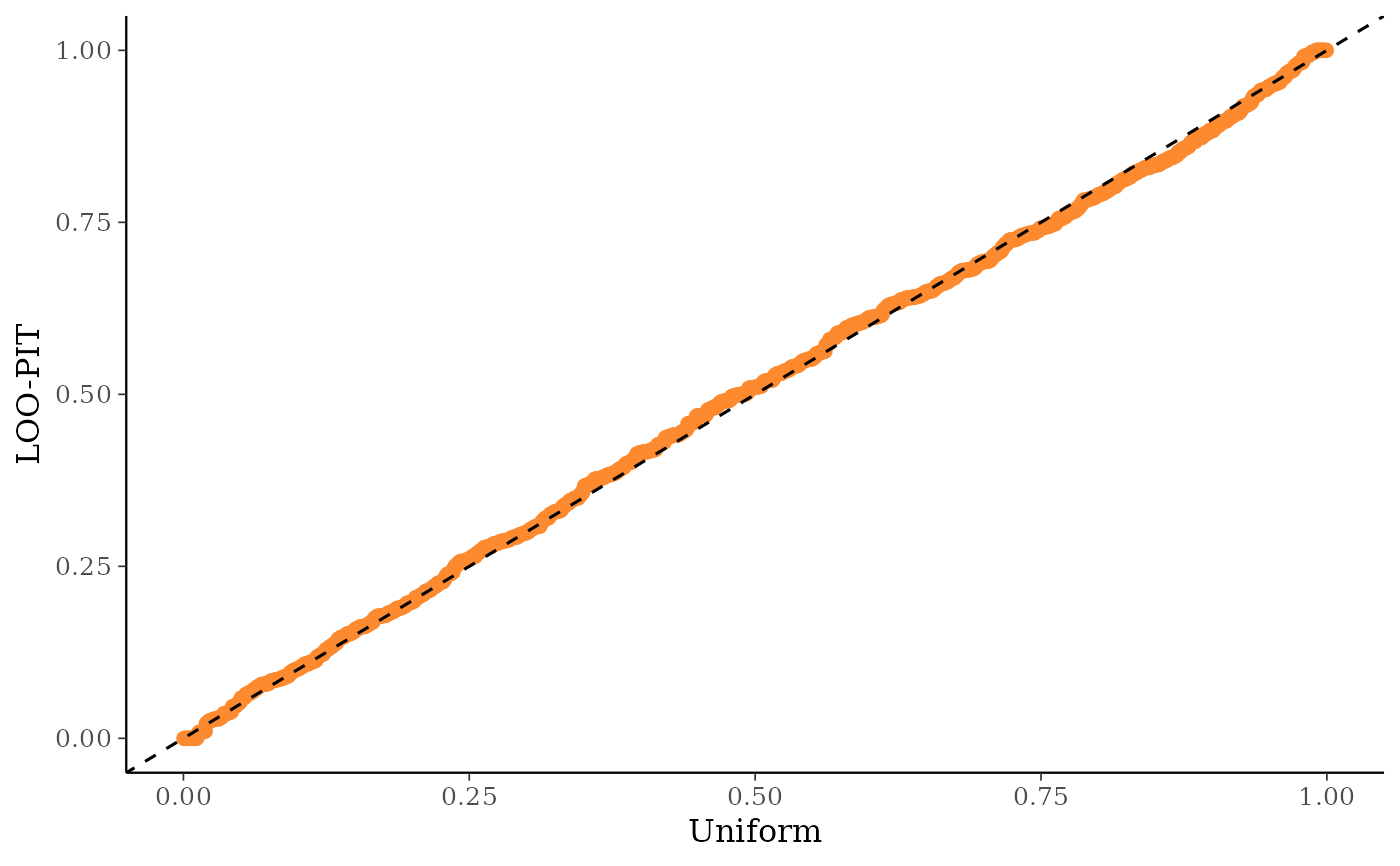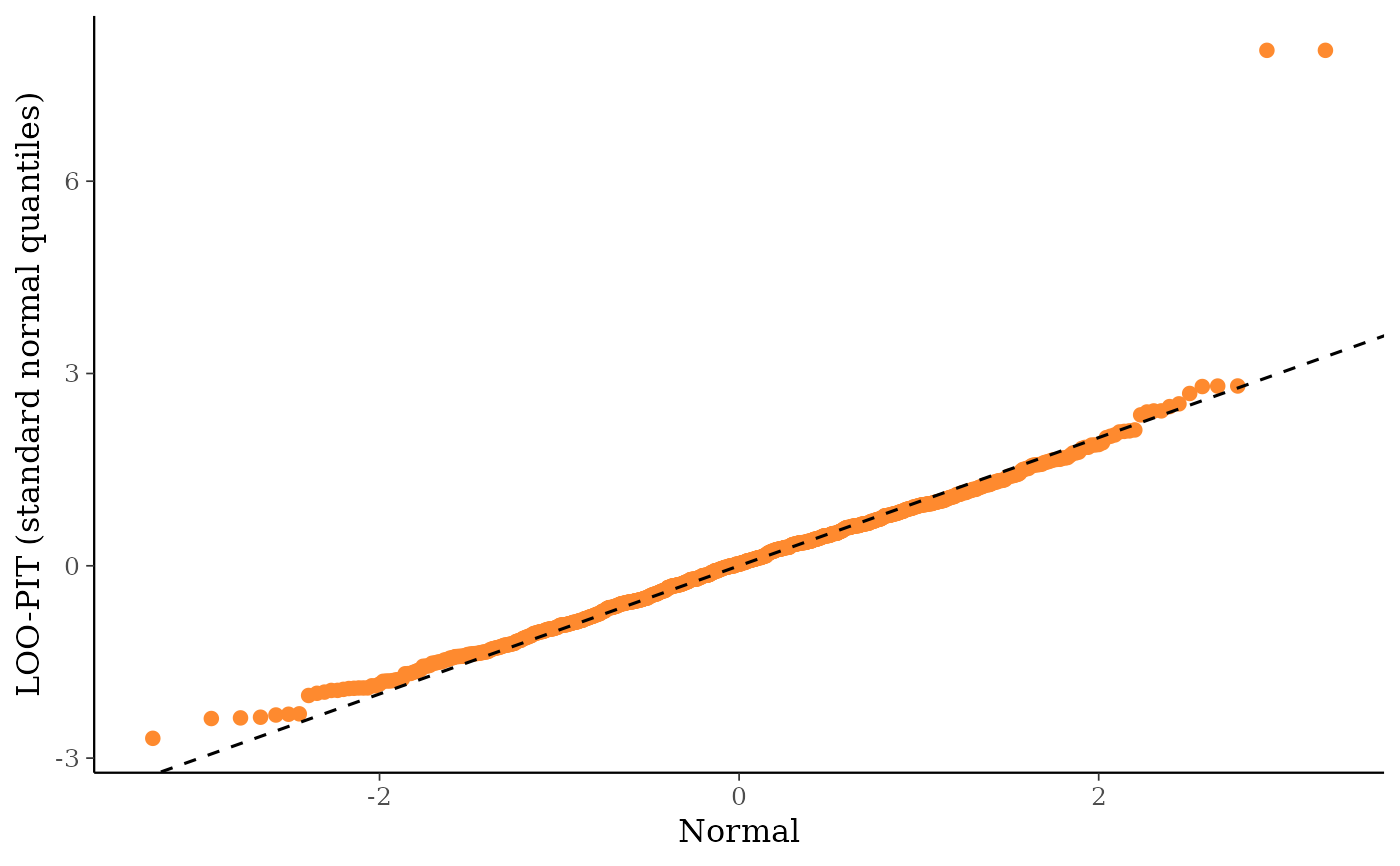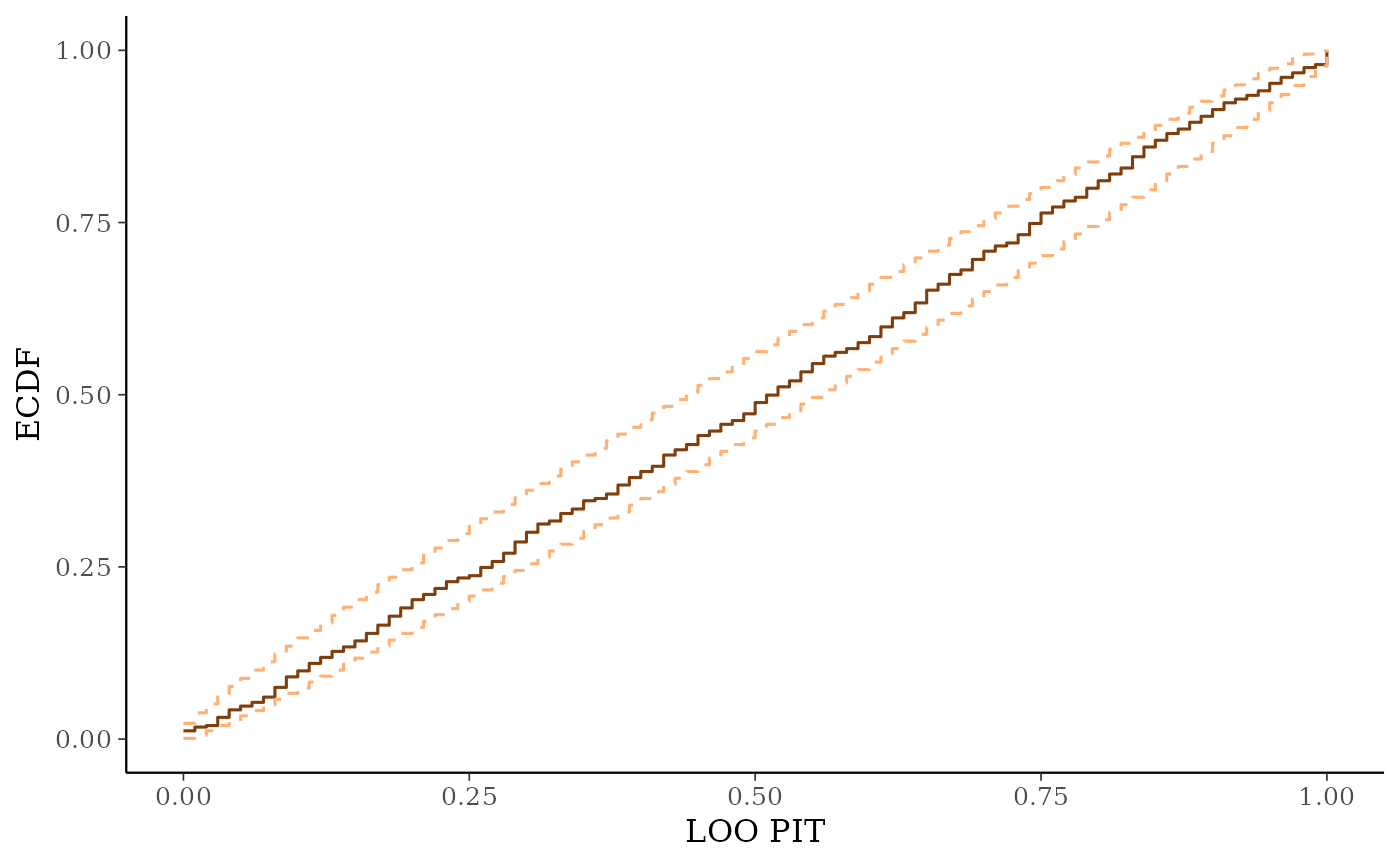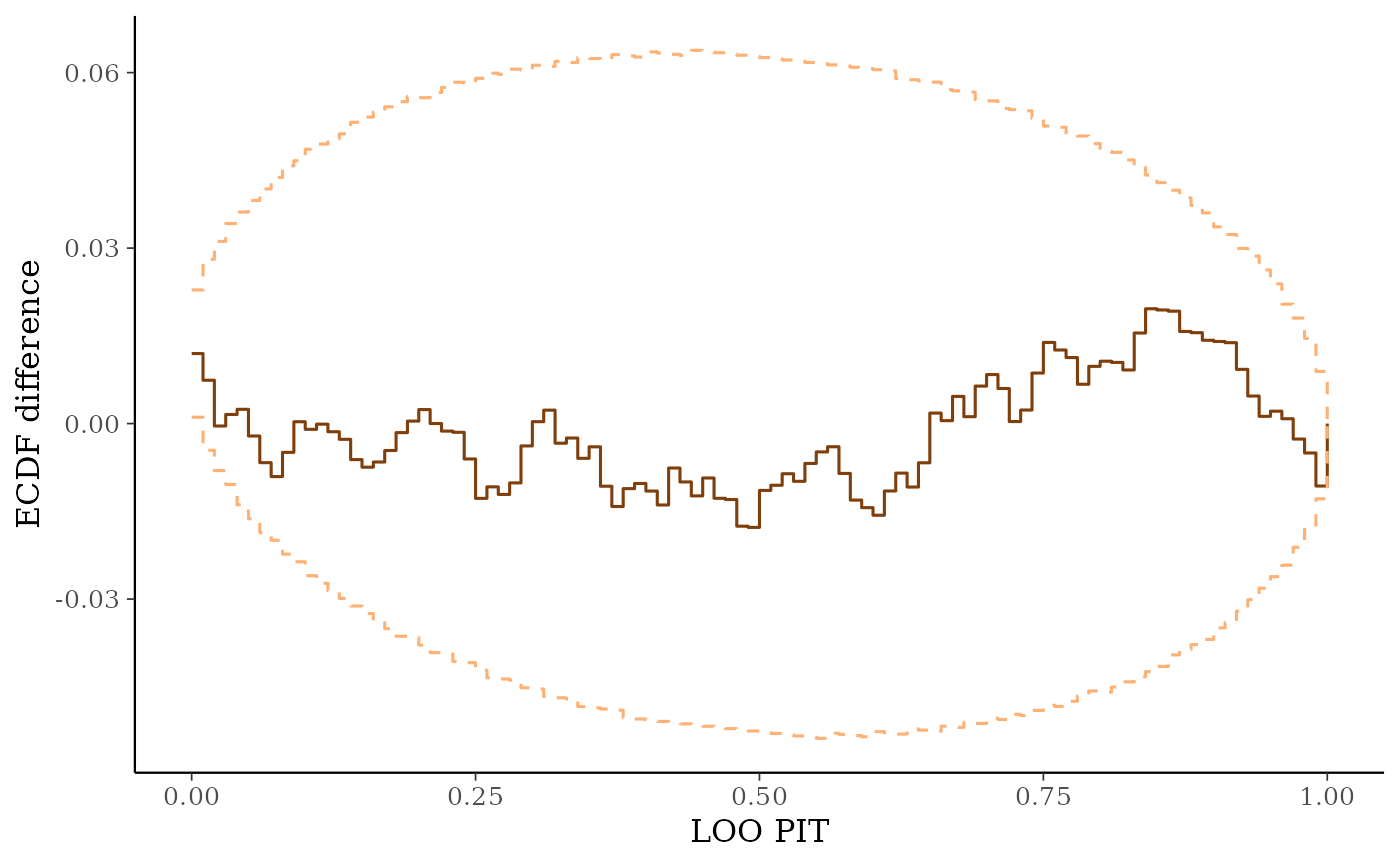Leave-One-Out (LOO) predictive checks. See the Plot Descriptions section, below, and Gabry et al. (2019) for details.

ppc_loo_pit_overlay(
y,
yrep,
lw,
...,
pit = NULL,
samples = 100,
size = 0.25,
alpha = 0.7,
boundary_correction = TRUE,
grid_len = 512,
bw = "nrd0",
trim = FALSE,
kernel = "gaussian",
n_dens = 1024
)

ppc_loo_pit_data(
y,
yrep,
lw,
...,
pit = NULL,
samples = 100,
bw = "nrd0",
boundary_correction = TRUE,
grid_len = 512
)

ppc_loo_pit_qq(
y,
yrep,
lw,
pit,
compare = c("uniform", "normal"),
...,
size = 2,
alpha = 1
)

ppc_loo_pit(
y,
yrep,
lw,
pit,
compare = c("uniform", "normal"),
...,
size = 2,
alpha = 1
)

ppc_loo_intervals(
y,
yrep,
psis_object,
subset = NULL,
intervals = NULL,
...,
prob = 0.5,
prob_outer = 0.9,
alpha = 0.33,
size = 1,
fatten = 2.5,
linewidth = 1,
order = c("index", "median")
)

ppc_loo_ribbon(
y,
yrep,
lw,
psis_object,
subset = NULL,
intervals = NULL,
...,
prob = 0.5,
prob_outer = 0.9,
alpha = 0.33,
size = 0.25
)

## Arguments

y

A vector of observations. See Details.

yrep

An S by N matrix of draws from the posterior (or prior) predictive distribution. The number of rows, S, is the size of the posterior (or prior) sample used to generate yrep. The number of columns, N is the number of predicted observations (length(y)). The columns of yrep should be in the same order as the data points in y for the plots to make sense. See the Details and Plot Descriptions sections for additional advice specific to particular plots.

lw

A matrix of (smoothed) log weights with the same dimensions as yrep. See loo::psis() and the associated weights() method as well as the Examples section, below.

...

Currently unused.

pit

For ppc_loo_pit_overlay() and ppc_loo_pit_qq(), optionally a vector of precomputed PIT values that can be specified instead of y, yrep, and lw (these are all ignored if pit is specified). If not specified the PIT values are computed internally before plotting.

samples

For ppc_loo_pit_overlay(), the number of data sets (each the same size as y) to simulate from the standard uniform distribution. The default is 100. The density estimate of each dataset is plotted as a thin line in the plot, with the density estimate of the LOO PITs overlaid as a thicker dark line.

alpha, size, fatten, linewidth

Arguments passed to code geoms to control plot aesthetics. For ppc_loo_pit_qq() and ppc_loo_pit_overlay(), size and alpha are passed to ggplot2::geom_point() and ggplot2::geom_density(), respectively. For ppc_loo_intervals(), size linewidth and fatten are passed to ggplot2::geom_pointrange(). For ppc_loo_ribbon(), alpha and size are passed to ggplot2::geom_ribbon().

boundary_correction

For ppc_loo_pit_overlay(), when set to TRUE (the default) the function will compute boundary corrected density values via convolution and a Gaussian filter, also known as the reflection method (Boneva et al., 1971). As a result, parameters controlling the standard kernel density estimation such as adjust, kernel and n_dens are ignored. NOTE: The current implementation only works well for continuous observations.

grid_len

For ppc_loo_pit_overlay(), when boundary_correction is set to TRUE this parameter specifies the number of points used to generate the estimations. This is set to 512 by default.

Optional arguments passed to stats::density() to override default kernel density estimation parameters. n_dens defaults to 1024.

trim

Passed to ggplot2::stat_density().

compare

For ppc_loo_pit_qq(), a string that can be either "uniform" or "normal". If "uniform" (the default) the Q-Q plot compares computed PIT values to the standard uniform distribution. If compare="normal", the Q-Q plot compares standard normal quantiles calculated from the PIT values to the theoretical standard normal quantiles.

psis_object

If using loo version 2.0.0 or greater, an object returned by the psis() function (or by the loo() function with argument save_psis set to TRUE).

subset

For ppc_loo_intervals() and ppc_loo_ribbon(), an optional integer vector indicating which observations in y (and yrep) to include. Dropping observations from y and yrep manually before passing them to the plotting function will not work because the dimensions will not match up with the dimensions of psis_object, but if all of y and yrep are passed along with subset then bayesplot can do the subsetting internally for y, yrep and psis_object. See the Examples section for a demonstration.

intervals

For ppc_loo_intervals() and ppc_loo_ribbon(), optionally a matrix of precomputed LOO predictive intervals that can be specified instead of yrep and lw (these are both ignored if intervals is specified). If not specified the intervals are computed internally before plotting. If specified, intervals must be a matrix with number of rows equal to the number of data points and five columns in the following order: lower outer interval, lower inner interval, median (50%), upper inner interval and upper outer interval (column names are ignored).

prob, prob_outer

Values between 0 and 1 indicating the desired probability mass to include in the inner and outer intervals. The defaults are prob=0.5 and prob_outer=0.9.

order

For ppc_loo_intervals(), a string indicating how to arrange the plotted intervals. The default ("index") is to plot them in the order of the observations. The alternative ("median") arranges them by median value from smallest (left) to largest (right).

## Value

A ggplot object that can be further customized using the ggplot2 package.

## Plot Descriptions

ppc_loo_pit_overlay(), ppc_loo_pit_qq()

The calibration of marginal predictions can be assessed using probability integral transformation (PIT) checks. LOO improves the check by avoiding the double use of data. See the section on marginal predictive checks in Gelman et al. (2013, p. 152--153) and section 5 of Gabry et al. (2019) for an example of using bayesplot for these checks.

The LOO PIT values are asymptotically uniform (for continuous data) if the model is calibrated. The ppc_loo_pit_overlay() function creates a plot comparing the density of the LOO PITs (thick line) to the density estimates of many simulated data sets from the standard uniform distribution (thin lines). See Gabry et al. (2019) for an example of interpreting the shape of the miscalibration that can be observed in these plots.

The ppc_loo_pit_qq() function provides an alternative visualization of the miscalibration with a quantile-quantile (Q-Q) plot comparing the LOO PITs to the standard uniform distribution. Comparing to the uniform is not good for extreme probabilities close to 0 and 1, so it can sometimes be useful to set the compare argument to "normal", which will produce a Q-Q plot comparing standard normal quantiles calculated from the PIT values to the theoretical standard normal quantiles. This can help see the (mis)calibration better for the extreme values. However, in most cases we have found that the overlaid density plot (ppc_loo_pit_overlay()) function will provide a clearer picture of calibration problems than the Q-Q plot.

ppc_loo_intervals(), ppc_loo_ribbon()

Similar to ppc_intervals() and ppc_ribbon() but the intervals are for the LOO predictive distribution.

Other PPCs: PPC-censoring, PPC-discrete, PPC-distributions, PPC-errors, PPC-intervals, PPC-overview, PPC-scatterplots, PPC-test-statistics

## Examples


# \dontrun{
suppressPackageStartupMessages(library(rstanarm))
suppressPackageStartupMessages(library(loo))

#> 1     1 AITKIN 0.83290912  -0.6890476
#> 2     0 AITKIN 0.83290912  -0.6890476
#> 3     0 AITKIN 1.09861229  -0.6890476
#> 4     0 AITKIN 0.09531018  -0.6890476
#> 5     0  ANOKA 1.16315081  -0.8473129
#> 6     0  ANOKA 0.95551145  -0.8473129
fit <- stan_lmer(
log_radon ~ floor + log_uranium + floor:log_uranium
+ (1 + floor | county),
iter = 1000,
chains = 2,
cores = 2
)
y <- radon$log_radon yrep <- posterior_predict(fit) loo1 <- loo(fit, save_psis = TRUE, cores = 4) #> Warning: Found 2 observation(s) with a pareto_k > 0.7. We recommend calling 'loo' again with argument 'k_threshold = 0.7' in order to calculate the ELPD without the assumption that these observations are negligible. This will refit the model 2 times to compute the ELPDs for the problematic observations directly. psis1 <- loo1$psis_object
lw <- weights(psis1) # normalized log weights

# marginal predictive check using LOO probability integral transform
color_scheme_set("orange")
ppc_loo_pit_overlay(y, yrep, lw = lw)
#> NOTE: The kernel density estimate assumes continuous observations and is not optimal for discrete observations.ppc_loo_pit_qq(y, yrep, lw = lw)ppc_loo_pit_qq(y, yrep, lw = lw, compare = "normal")
#> Warning: NaNs produced
#> Warning: Removed 5 rows containing non-finite values (stat_qq()).
#> Warning: Removed 5 rows containing non-finite values (stat_qq_line()).# loo predictive intervals vs observations
keep_obs <- 1:50
ppc_loo_intervals(y, yrep, psis_object = psis1, subset = keep_obs)color_scheme_set("gray")
ppc_loo_intervals(y, yrep, psis_object = psis1, subset = keep_obs,
order = "median")# }